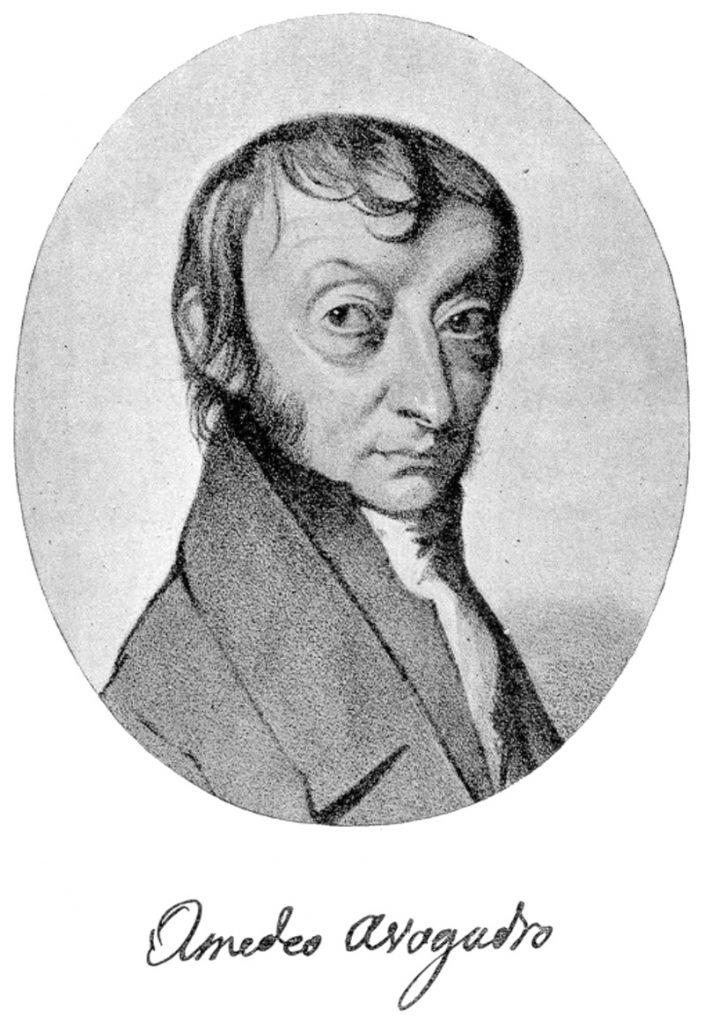Science Site

Published on Jun 1, 2016

Tags:Keywords:, ,During the 19th century and at the beginning of the 20th century, several of the principles of chemistry that are commonplace now were being discovered, discussed and formulated by the scientists. One of those was principles was put forward by the Italian scientist Amedeo Avogadro. Avogadro suggested that if two gases occupied the same volume at a given temperature and pressure then they must have the same number of particles, irrespective of the nature or chemical properties that those gases exhibited. After the concept of mole came into picture, scientists observed that one mole of any substance contained approximately 6.022 x 1023 particles of that substance. This number was called Avogadro’s number to honor the work of the Italian scientist.

What should be the standard?

Initially, some scientists used the mass of hydrogen as the standard for calculating masses of other elements and said that one molecule of the gas contained 2g of hydrogen. However, other scientists like the idea of using oxygen as the standard because oxygen formed oxides with a large number of elements and so it would have been easier to determine the mass those compounds if oxygen was used as a standard. The problem arose with the introduction of isotopes. Oxygen as three isotopes and chemists decided to give the average mass of an oxygen atom the value of 16g. This average mass is calculated by multiplying the abundance of an isotope in nature with the molar mass that is associated with it. This caused a problem for the physicists who dealt with O16 only and not with O17 or O18. In order to resolve the issue, it was decided that C12 or carbon-12 will be used as a standard because it was almost mid-way between hydrogen and oxygen standards.

How does Avogadro’s number help scientists?

Avogadro’s number establishes a connection between the mass that a sample of substance has with the molar mass of the substance. Mole is a different way of representing Avogadro’s number just like a dozen is another way of denoting 12 parts of something. Avogadro’s number helps in determining the number of particles of a particular entity, be like atoms or molecules. Mass of one mole of C -12 atoms is 12g. A molar amount of C-12 will contain 6.022 x 1023 atoms. Thus, Avogadro’s number forms a link between the microscopic world of atoms to the macroscopic world of grams and kilograms in which we conduct our calculations.